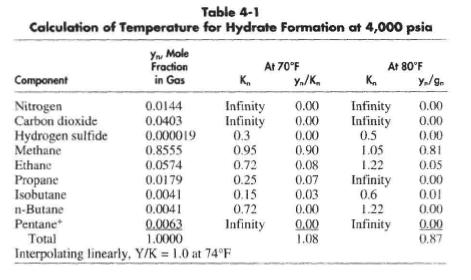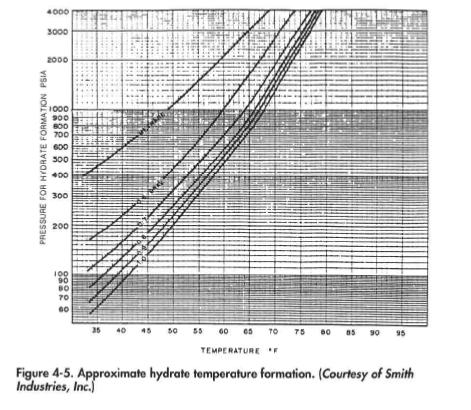# Determination of Hydrate Formation Temperature or Pressure

Knowledge of the temperature and pressure of a gas stream at the wellhead is important for determining whether hydrate formation can be expected when the gas is expanded into the flow lines. The temperature at the wellhead can change as the reservoir conditions or production rate changes over the producing life of the well. Thus, wells that initially flowed at conditions at which hydrate formation in downstream equipment was not expected may eventually require hydrate prevention, or vice versa.

If the composition of the stream is known, the hydrate temperature can be predicted using vapor-solid (hydrate) equilibrium constants. The basic equation for this prediction is:The vapor-solid equilibrium constant is determined experimentally and is defined as the ratio of the mol fraction of the hydrocarbon component in gas on a water-free basis to the mol fraction of the hydrocarbon component in the solid on a water-free basis. That is:Graphs giving the vapor-solid equilibrium constants at various temperatures and pressures are given in Figures 4-1 through 4-4. For nitrogen and components heavier than butane, the equilibrium constant is taken as infinity.

The steps for determining the hydrate temperature at a given system pressure are as follows: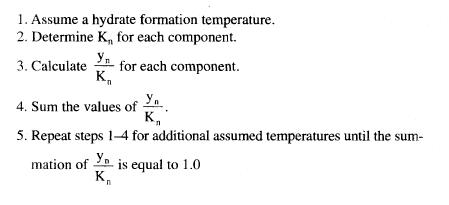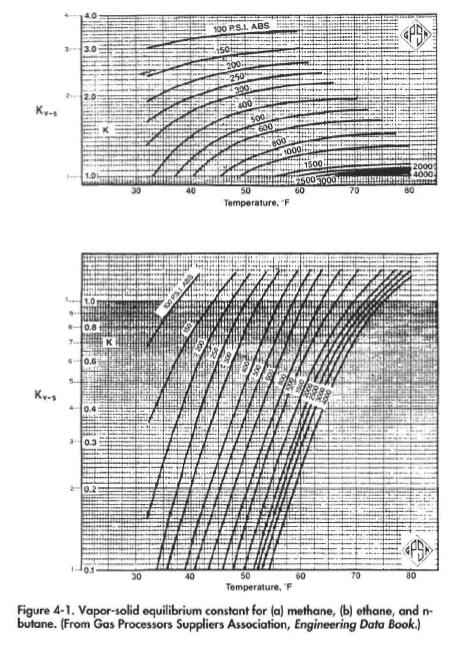The presence of H2S should not be overlooked in the determination of susceptibility of a gas to form hydrates. At concentrations of 30% or greater, hydrates will form in hydrocarbon gases at about the same temperature as in pure H2S.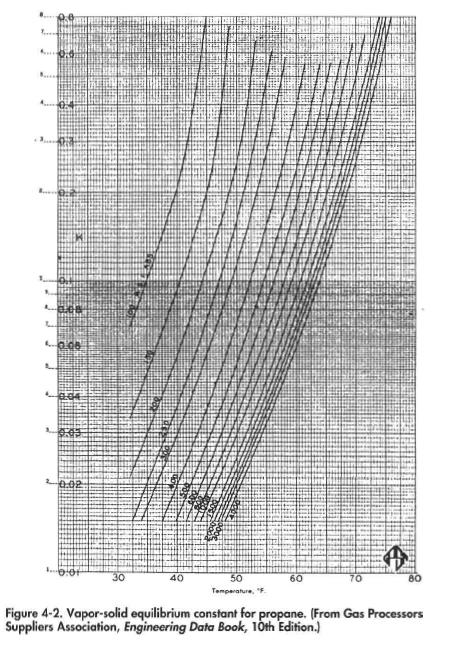Table 4-1 is an example calculation of the temperature below which hydrates will form at the 4,000 psia flowing temperature for the example gas composition of Table 1-1. From this calculation, hydrates will form at temperatures below 74°F.If the gas composition is not known, this procedure cannot be used to develop the hydrate formation point. Figure 4-5 gives approximate hydrate formation temperatures as a function of gas gravity and pressure. For example, for the 0.67 specific gravity gas of our example field (Table 2-10), Figure 4-5 predicts a hydrate formation temperature at 4,000 psia at 76°F.